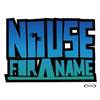• 254 Vote(s) - 2.8 Average
• 1
• 2
• 3
• 4
• 508-29-2011, 09:51 AM,thiguchiJunior MemberReputation: 2 Posts: 8 Joined: Jul 2011
ŃüōŃéōŃü½ŃüĪŃü»ŃĆéĶ│¬ÕĢÅŃü¦ŃüÖ’╝ü

ŃéłŃéŹŃüŚŃüÅŃüŖķĪśŃüäŃüŚŃüŠŃüÖ’╝ü

Code:
{import * from CURL.GUI.CHARTS}
{let records:RecordSet =
{RecordSet
{RecordFields
{RecordField "KPH", caption = "Kilometers/hour", domain = int},
{RecordField "TD", caption = "Thinking distance", domain = int},
{RecordField "BD", caption = "Braking distance", domain = int},
{RecordField "OD", caption = "Overall distance", domain = int}
},
{RecordData KPH = 32, TD = 6, BD = 6, OD = 12},
{RecordData KPH = 48, TD = 9, BD = 14, OD = 23},
{RecordData KPH = 64, TD = 12, BD = 24, OD = 36},
{RecordData KPH = 80, TD = 15, BD = 38, OD = 53},
{RecordData KPH = 97, TD = 18, BD = 55, OD = 73},
{RecordData KPH = 113, TD = 21, BD = 75, OD = 96}
}
}
{let chart:LayeredChart =
{LayeredChart
width = 15cm,
height = 6cm,
plot-area = {LayeredPlotArea
right-axis-grid-lines? = true
},
left-axis =
{ChartAxis
{ChartDataSeries records, "OD"},
axis-label = "OD"
},
right-axis =
{ChartAxis
{ChartDataSeries records, "BD"},
axis-label = "BD"
},
bottom-axis =
{ChartAxis
{ChartDataSeries records, "KPH"},
force-zero? = false
},
{LineLayer
{ChartDataSeries records, "OD"},
{ChartDataSeries records, "BD"},
{ChartDataSeries records, "TD"}
}
}
}
{VBox
{RecordGrid
height = 4cm,
width = 13cm,
record-source = records
},
chart
}
08-29-2011, 02:11 PM,c-sCurl EngineerReputation: 11 Posts: 57 Joined: Jul 2011
ŃüōŃüåŃüÖŃéīŃü░Õ”éõĮĢŃü¦ŃüŚŃéćŃüåŃüŗŃĆé

Code:
{curl 7.0, 8.0 applet}

{import * from CURL.GUI.CHARTS}

{define-class public open MyLayeredPlotArea {inherits LayeredPlotArea}

{constructor public {default
grid-line-group:Shape = {ShapeGroup},
left-grid-line-group:Shape = {ShapeGroup},
right-grid-line-group:Shape = {ShapeGroup},
top-grid-line-group:Shape = {ShapeGroup},
bottom-grid-line-group:Shape = {ShapeGroup},
color:FillPattern = FillPattern.silver,
...
}
{construct-super
grid-line-group = grid-line-group,
left-grid-line-group = left-grid-line-group,
right-grid-line-group = right-grid-line-group,
top-grid-line-group = top-grid-line-group,
bottom-grid-line-group = bottom-grid-line-group,
color = color,
...
}
}

{method protected open {generate-grid-lines
container:Shape,
axis:ChartAxis,
horizontal?:bool
}:void
{if axis.position == AxisPosition.right then
{for tick in {axis.get-major-ticks-Iterator} do
let constant pos:Distance = tick.position
let p1:Distance2d = {Distance2d 0m, 0m}
let p2:Distance2d = {Distance2d 0m, 0m}
set p1 = {Distance2d -self.width, pos}
set p2 = {Distance2d 0m, pos}
set p1 = {axis.transform-to-chart p1}
set p2 = {axis.transform-to-chart p2}
set p1 = {self.transformation.inverse-transform-point p1}
set p2 = {self.transformation.inverse-transform-point p2}

{container.add {PathShape {Path p1, PathOperation.line-to, p2}}}
}
else
{super.generate-grid-lines
container,
axis,
horizontal?
}
}
}

}

{let records:RecordSet =
{RecordSet
{RecordFields
{RecordField "KPH", caption = "Kilometers/hour",   domain = int},
{RecordField "TD",  caption = "Thinking distance", domain = int},
{RecordField "BD",  caption = "Braking distance",  domain = int},
{RecordField "OD",  caption = "Overall distance",  domain = int}
},
{RecordData KPH =  32, TD =  6, BD =  6, OD = 12},
{RecordData KPH =  48, TD =  9, BD = 14, OD = 23},
{RecordData KPH =  64, TD = 12, BD = 24, OD = 36},
{RecordData KPH =  80, TD = 15, BD = 38, OD = 53},
{RecordData KPH =  97, TD = 18, BD = 55, OD = 73},
{RecordData KPH = 113, TD = 21, BD = 75, OD = 96}
}
}

{LayeredChart
width = 15cm,
height = 6cm,
plot-area =
{MyLayeredPlotArea
left-axis-grid-lines? = false,
right-axis-grid-lines? = true
},
left-axis =
{ChartAxis
{ChartDataSeries records, "OD"},
axis-label = "OD"
},
right-axis =
{ChartAxis
{ChartDataSeries records, "BD"},
axis-label = "BD"
},
bottom-axis =
{ChartAxis
{ChartDataSeries records, "KPH"},
force-zero? = false
},
{LineLayer
{ChartDataSeries records, "OD"},
{ChartDataSeries records, "BD"},
{ChartDataSeries records, "TD"}
}
}
 « Next Oldest | Next Newest »

Forum Jump:

Users browsing this thread:
1 Guest(s)

MyBB SQL Error

MyBB has experienced an internal SQL error and cannot continue.

SQL Error:
1017 - Can't find file: 'mybb_threadviews' (errno: 2)
Query:
INSERT INTO mybb_threadviews (tid) VALUES('250')